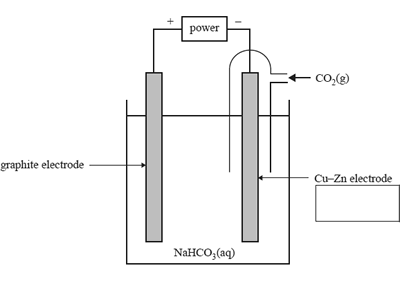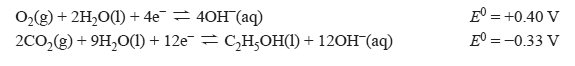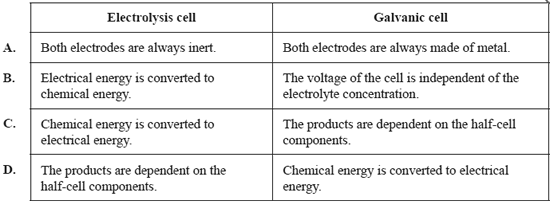Electrolytic cells(2020 VCE) 1) The electrolysis of carbon dioxide gas, CO2, in water is one way of making ethanol, C2H5OH. The diagram below shows a CO2–H2O electrolysis cell. The electrolyte used in the electrolysis cell is sodium bicarbonate solution, NaHCO3(aq).The following half-cell reactions occur in the CO2–H2O electrolysis cell.a. Identify the Cu–Zn electrode as either the anode or the cathode in the box provided in the diagram above. b. Determine the applied voltage required for the electrolysis cell to operate. c. Write the balanced equation for the overall electrolysis reaction. d. Identify the oxidising agent in the electrolysis reaction. Give your reasoning using oxidation numbers. Solution e. A current of 2.70 A is passed through the CO2–H2O electrolysis cell. The cell has an efficiency of 58%. Calculate the time taken, in minutes, for this cell to consume 6.05 × 10-3 mol of CO2(g). Solution2) Which one of the following pairs of statements is correct for both electrolysis cells and galvanic cells?Solution